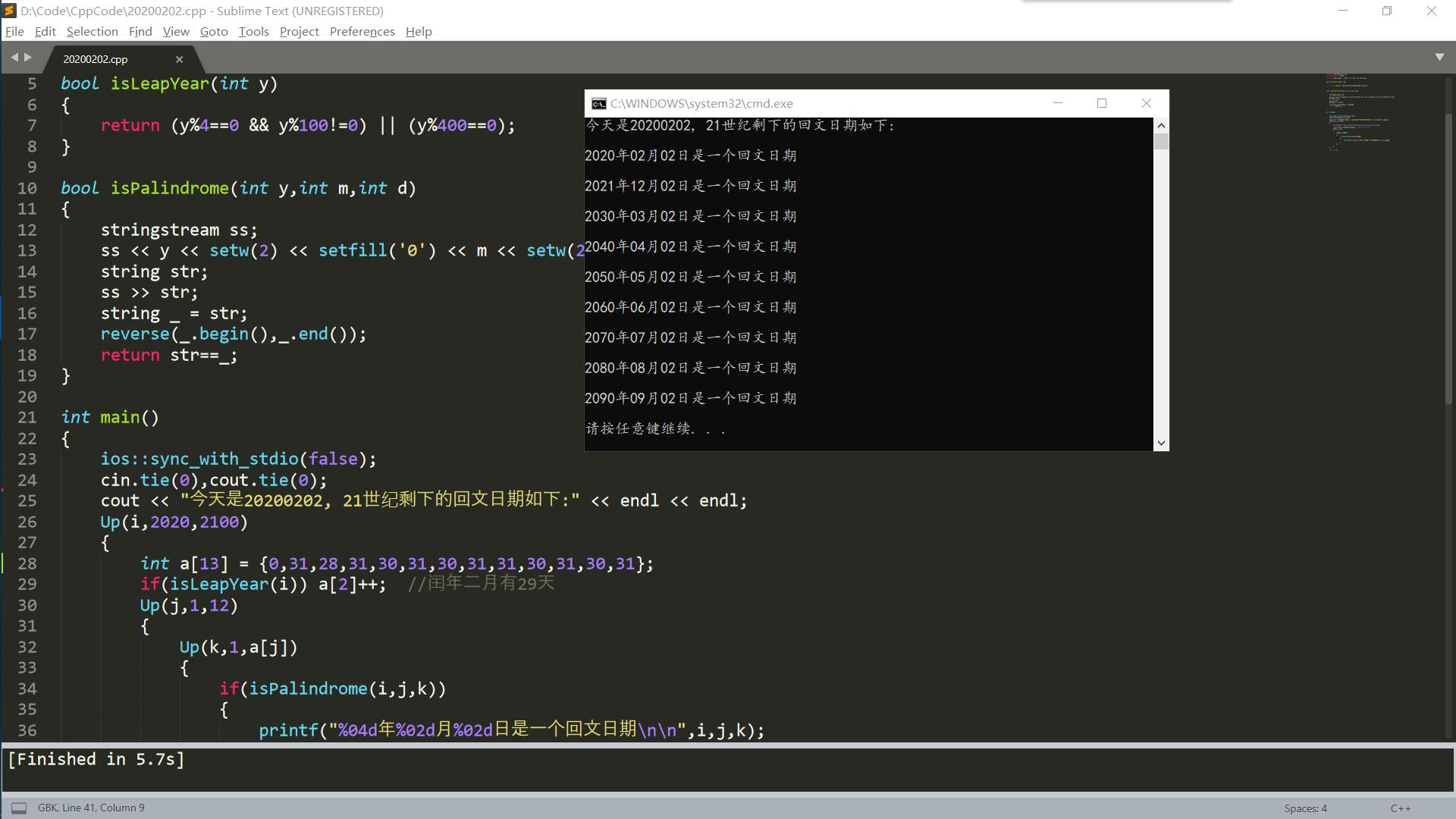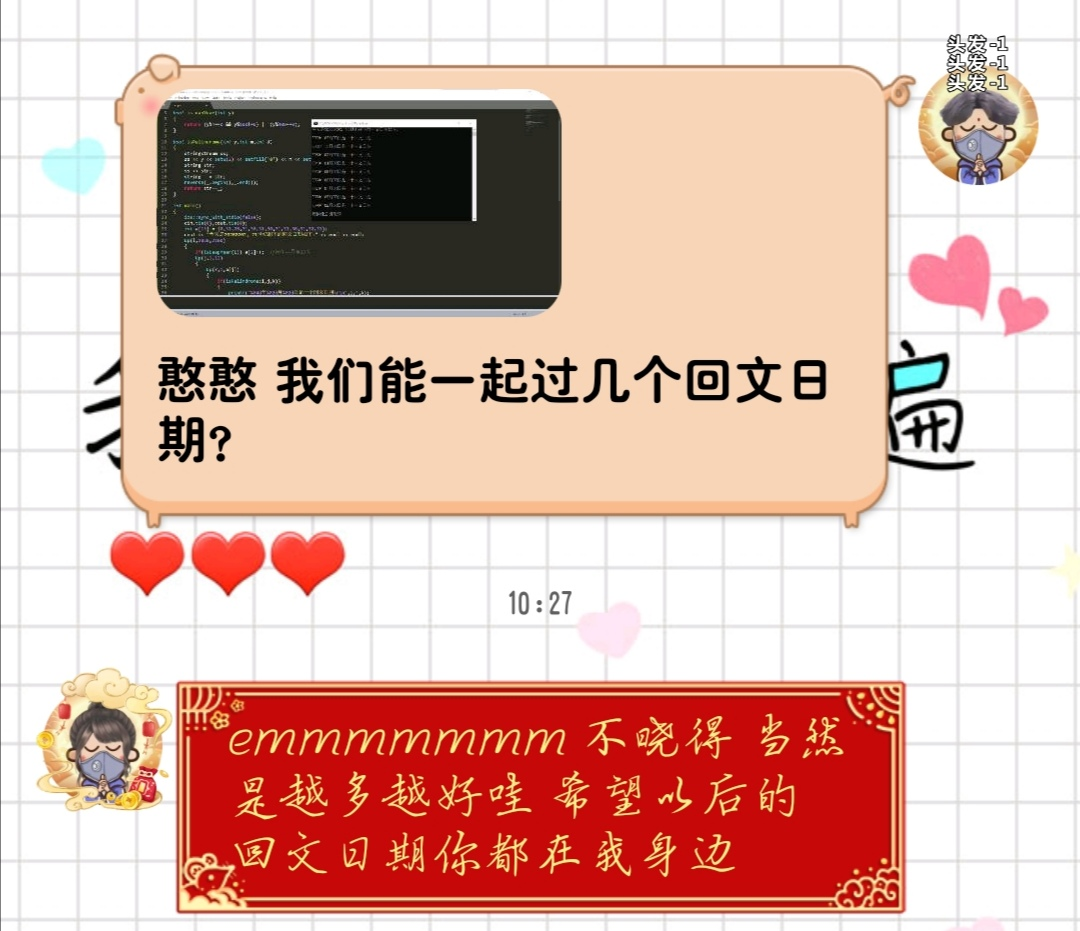### 写在前面：### 源代码：

``````#include <bits/stdc++.h>
using namespace std;
#define Up(i,a,b) for(int i = a; i <= b; i++)

bool isLeapYear(int y)
{
return (y%4==0 && y%100!=0) || (y%400==0);
}

bool isPalindrome(int y,int m,int d)
{
stringstream ss;
ss << y << setw(2) << setfill('0') << m << setw(2) << setfill('0') << d;
string str;
ss >> str;
string _ = str;
reverse(_.begin(),_.end());
return str==_;
}

int main()
{
ios::sync_with_stdio(false);
cin.tie(0),cout.tie(0);
cout << "今天是20200202, 21世纪剩下的回文日期如下:" << endl << endl;
Up(i,2020,2100)
{
int a = {0,31,28,31,30,31,30,31,31,30,31,30,31};
if(isLeapYear(i)) a++;  //闰年二月有29天
Up(j,1,12)
{
Up(k,1,a[j])
{
if(isPalindrome(i,j,k))
{
printf("%04d年%02d月%02d日是一个回文日期\n\n",i,j,k);
}
}
}
}
return 0;
}``````

### 运行界面：